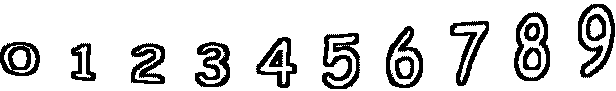2 - 0 =        1 - 1 =7 - 1 =        8 - 6 =        0 - 0 =        7 - 1 = 0 - 0 =        0 - 0 =        2 - 0 =        7 - 7 =